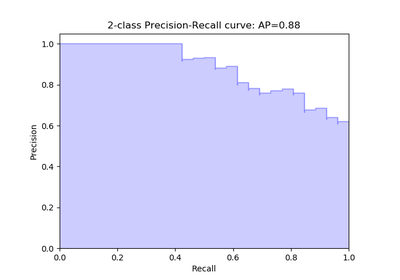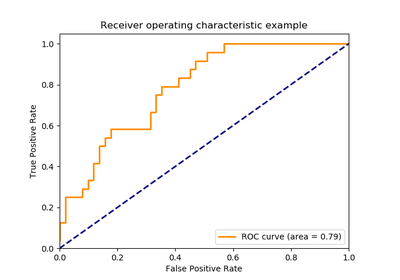# `sklearn.preprocessing`.label_binarize¶

`sklearn.preprocessing.``label_binarize`(y, classes, neg_label=0, pos_label=1, sparse_output=False)[source]

Binarize labels in a one-vs-all fashion

Several regression and binary classification algorithms are available in the scikit. A simple way to extend these algorithms to the multi-class classification case is to use the so-called one-vs-all scheme.

This function makes it possible to compute this transformation for a fixed set of class labels known ahead of time.

Parameters: y : array-like Sequence of integer labels or multilabel data to encode. classes : array-like of shape [n_classes] Uniquely holds the label for each class. neg_label : int (default: 0) Value with which negative labels must be encoded. pos_label : int (default: 1) Value with which positive labels must be encoded. sparse_output : boolean (default: False), Set to true if output binary array is desired in CSR sparse format Y : numpy array or CSR matrix of shape [n_samples, n_classes] Shape will be [n_samples, 1] for binary problems.

`LabelBinarizer`
class used to wrap the functionality of label_binarize and allow for fitting to classes independently of the transform operation

Examples

```>>> from sklearn.preprocessing import label_binarize
>>> label_binarize([1, 6], classes=[1, 2, 4, 6])
array([[1, 0, 0, 0],
[0, 0, 0, 1]])
```

The class ordering is preserved:

```>>> label_binarize([1, 6], classes=[1, 6, 4, 2])
array([[1, 0, 0, 0],
[0, 1, 0, 0]])
```

Binary targets transform to a column vector

```>>> label_binarize(['yes', 'no', 'no', 'yes'], classes=['no', 'yes'])
array([,
,
,
])
```

## Examples using `sklearn.preprocessing.label_binarize`¶Precision-Recall